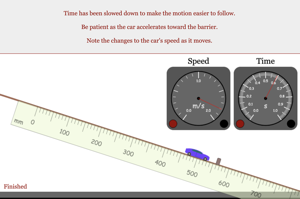Programs for Programs for Equations of Motion
Below are all the programs that might be used with this topic. Click on "See Resources" to see if there are any student directions, lab sheets or other materials that have been created for this program.
Equations of Motion Lab

Lab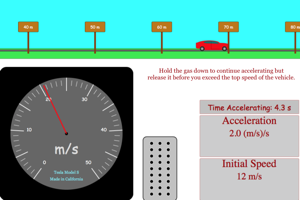Car Acceleration (No Forces)

Homework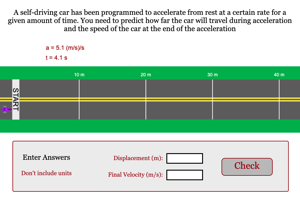Father Brown Constant Acceleration Lab

Lab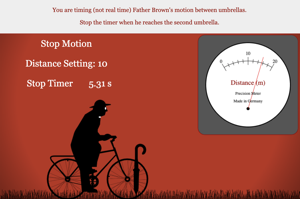Two Stages of Motion Problem 1

Homework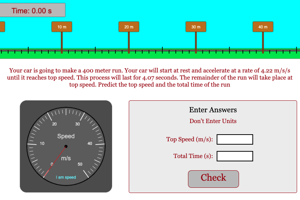Two Stages of Motion Problem 2

Homework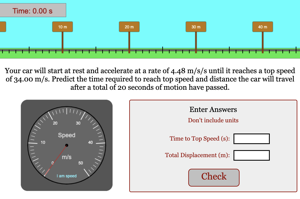Accelerated Motion Problem 1

HomeworkAccelerated Motion Problem 2

Homework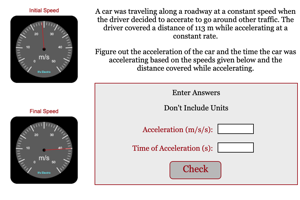Initial Speed from Stopping Distance

Homework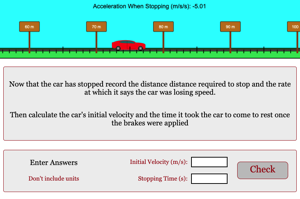Acceleration on Incline with Prediction Lab

Lab